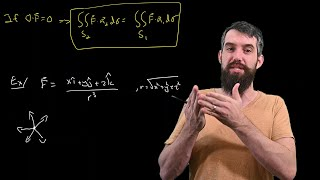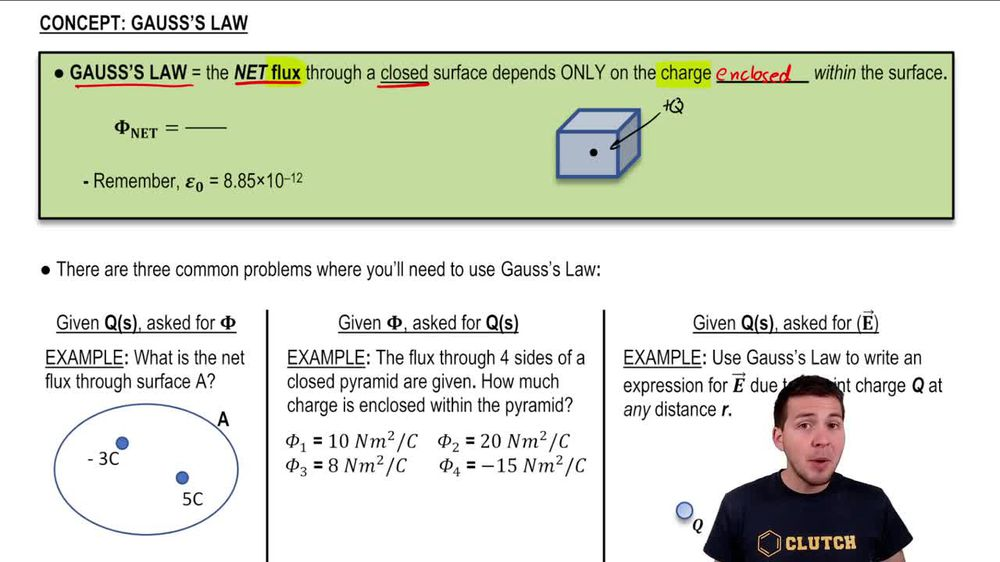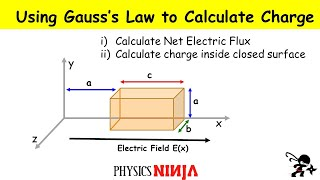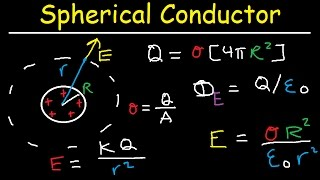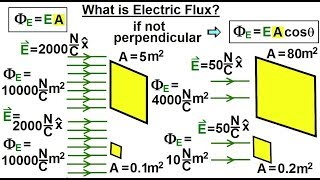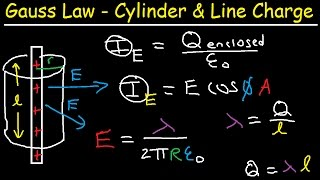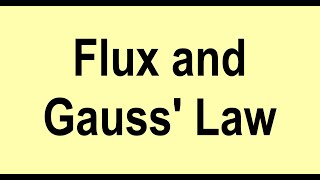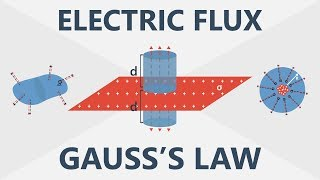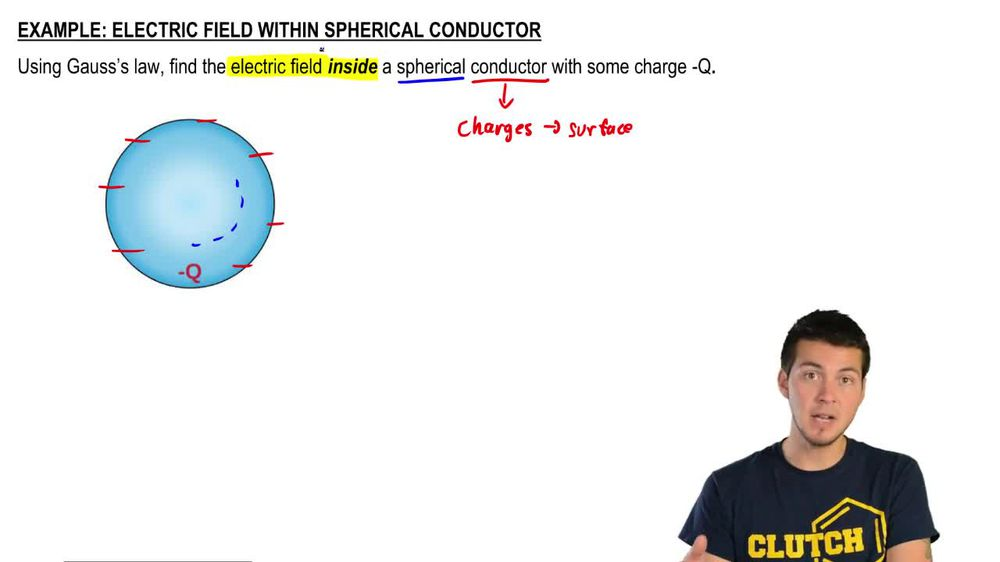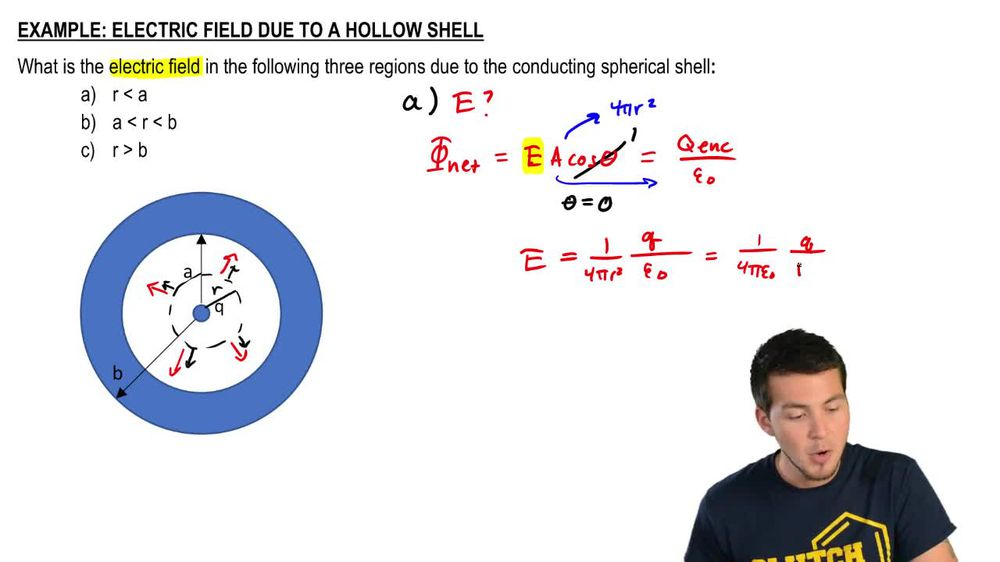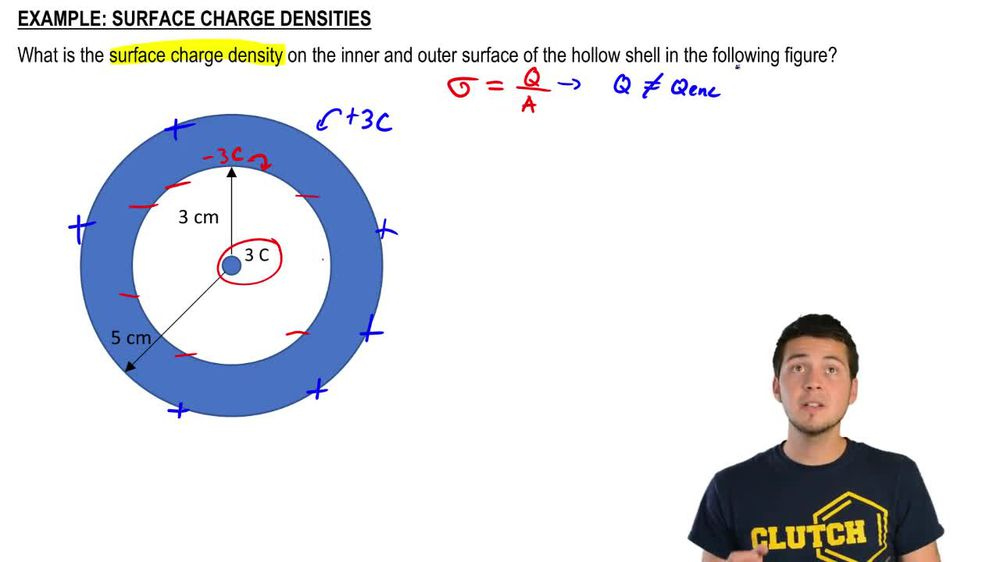Start typing, then use the up and down arrows to select an option from the list.
1. 24. Electric Force & Field; Gauss' Law2. Gauss' Law# Electric Field Within Spherical Conductor

by Patrick Ford
181 views
5
2
5
What's up, guys? Let's gets more practice with Gasses Law by checking out this example together. So we're supposed to use gas Is law to figure out what the electric field is inside of a spherical conductor with some charge? That's negative. Q. Okay, so what I want to point out is that this conductor here remember that the property of a conductor means that any net charges will always move and distribute themselves along the surface of that conductor. So if you have some conductor right here and it has some net charge, negative Q. And basically all of these negative charges will always distribute themselves evenly among that surface because they want to maximize their distance away from each other. So now we also know what we're working with. The third type of problem of gasses law figuring out what the electric field is. So we have to choose a Gaussian surface with symmetry. Now, if you're working with a spherical sort of like conductor or spherical geometry thing, the best thing that you're gonna use is also a sphere. But we have to use this field. We have to figure what the field is inside of this conductor. So the Gaussian surface that I'm gonna choose right here is gonna be within the conductor. Okay, So, basically, how do we relate this gas is law to the electric field. We have that the total flux, which is e a times the cosine of theta is equal to the Cuban closed, so I can either use big cure Little Q Q closed over Epsilon. Not now. What happens here? I've had this surface. I've chosen this Gaussian surface within this conductor, and it's it's, um, arbitrary distance. So I'm choosing it could be here or here, over here somewhere. And I have to figure out what the enclosed amount of charge is now. We said that for a conductor, all of the charges, all the net charges will always distribute themselves evenly among the surface. So that means that the amount of charge that's enclosed within the surface here is zero. There is no charges here. There's no in charges within this Gaussian surface that I've done. There is a charge if I chosen this Gaussian surface outside, but that's not what we're asked, were asked to figure out what the inside electric field is, so that means that this whole entire term is just equal to it's just equal to zero because Q enclosed is equal to zero. Now what happens is that what does that mean for the electric field? What We know that the area is not equal to zero because the area of our gas and surface is just gonna be whatever we choose that surface to be. It could be. It's, um, Radius are something like that. So what that means is that the electric field has to be equal to zero, and that actually makes sense. Think about when we're talking about the electric fields inside of conductors. We said the electric field inside a conductor always has to be zero. So the gas is law actually helps reinforce this idea that if there are no charges within this Gaussian surface, then that means the electric field has to be zero. And that is the power Gasol's law. Let me know if you guys have any questions with this